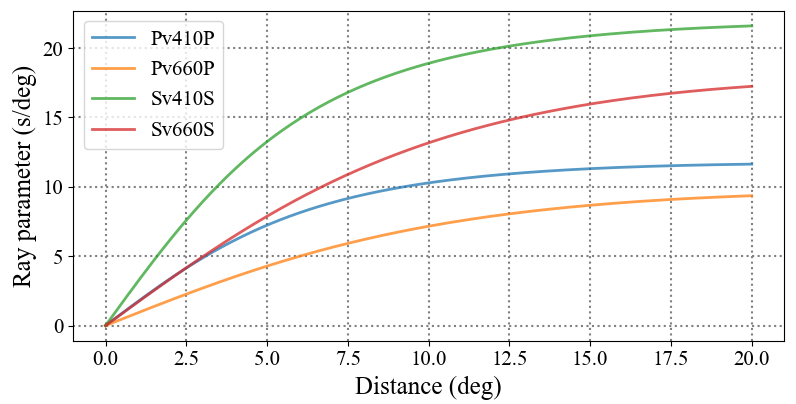Usually, we need to do back-projection from our back-azimuth $–$ slowness panel of body waves to geographic locations. We’ll obtain the location of some body phase if we know the ray parameter of the body phase of corresponding epicentral distance. Here, we show you how to calculate the ray parameters versus the epicentral distances using the python library obspy.

  1 2 3 4 5 6 7 8 9 10 11 12 13 14 15 16 17 18 19 20 21 22 23 24 25 26 27  from obspy.taup import TauPyModel import matplotlib.pyplot as plt import numpy as np model = TauPyModel(model='prem') dg = 0.1 slow = [[i*dg for i in range(201)]] phase1 = ['Pv410P', 'Pv660P', 'Sv410S', 'Sv660S'] phase2 = ['PcP', 'PcS', 'ScS', 'PKiKP', 'PKiKS', 'SKiKS'] for ph in phase1: tmp = [] for i in range(201): arrivals = model.get_ray_paths(0, i*dg, phase_list=[ph]) tmp.append(arrivals.ray_param_sec_degree) slow.append(tmp) SLOW = np.array(slow) plt.figure(figsize=(5, 7)) for i, ph in enumerate(phase1): plt.plot(SLOW, SLOW[i+1], alpha=0.75, label=ph) plt.legend() plt.xlabel('Distance (deg)') plt.ylabel('Ray parameter (deg/s)') plt.grid(ls=':', lw=1.5, color='gray', zorder=0) plt.tight_layout() plt.show()